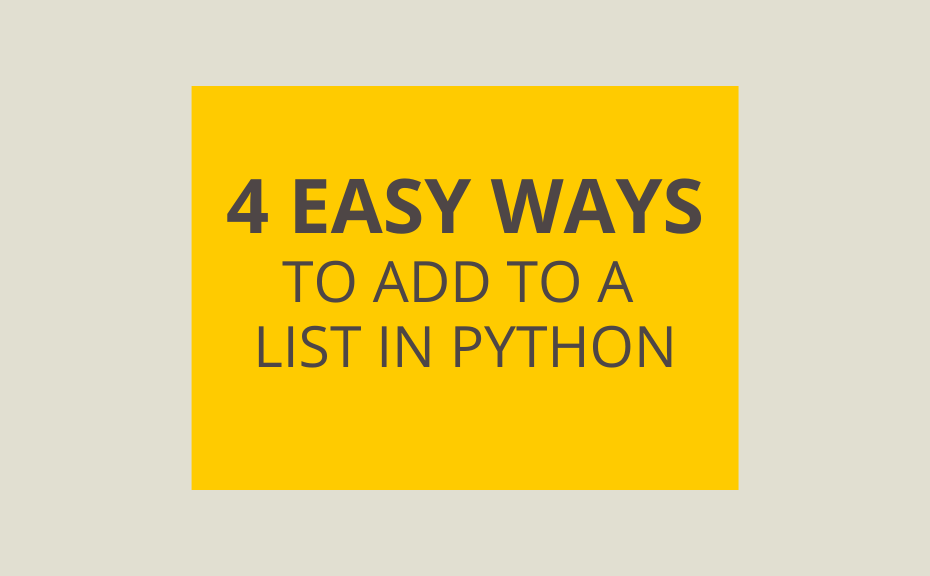# How to Append to Lists in Python – 4 Easy Methods!Lists are an incredibly useful feature in Python! In this post, we’ll explore how to append to lists in Python. We’ll cover this in more detail in the post below, but here’s a quick overview of how to append to lists in Python.

• append() – appends an object to the end of a list
• insert() – inserts an object before a provided index
• extend() – append items of iterable objects to end of a list
• + operator – concatenate multiple lists together

## A Quick Overview of Lists in Python

Lists in Python are mutable, meaning they can be changed after being defined. They can also contain different data types, including strings, integers, floats, and even other lists!

Lists in Python are indexed and have a defined sequence when initialized. The elements are indexed beginning at 0. They can also be indexed with negative indices, beginning at -1.

To given an example of this, let’s create a list and access its first and its last item:

``````sample_list = ['datagy', 4, 100.0]
print('First item: ',sample_list)
print('Last item: ',sample_list[-1])``````

This returns:

``````datagy
100.0``````

If you want to check out our tutorial on List Comprehensions, check out this link.

## Append to Lists in Python

The append() method adds a single item to the end of an existing list in Python. The method takes a single parameter and adds it to the end. The added item can include numbers, strings, lists, or dictionaries. Let’s try this out with a number of examples.

### Appending a Single Value to a List

Let’s add a single value to a list:

``````sample_list = [1,2,3]
sample_list.append(4)
print(sample_list)``````
``[1, 2, 3, 4]``

### Appending to List to a List

``````sample_list = [1,2,3]
sample_list2 = [4,5,6]
sample_list.append(sample_list2)
print(sample_list)``````
``[1, 2, 3, [4, 5, 6]]``

You’ll notice that the output’s fourth item is a separate list. If we tried to access the fourth item, we would output a list:

``print(sample_list)``
``[4, 5, 6]``

If we wanted to access the number 4 – the first value of the fourth item of sample_list – we would have to write the following code:

``print(sample_list)``
``4``

## Insert to List in Python

While the append method will always add an item to the end of a list, we can define where we would want an item added using the insert method.

The insert method takes two parameters:

1. Index: the index position to where the item should be added
2. Element: the item to be added to the list

Let’s try this out with an example:

``````sample_list = [1,2,3]
sample_list.insert(2,4)
print(sample_list)``````
``[1, 2, 4, 3]``

Check out some other Python tutorials on datagy, including our tutorials on Pivot Tables in Pandas and All You Need to Know about For Loops in Python.

We can also insert items using a negative index. The key thing to note here is that the index of -1 would insert the item in the second last spot:

``````sample_list = [1,2,3,4,5,6]
sample_list.insert(-1,99)
print(sample_list)``````
``[1, 2, 3, 4, 5, 99, 6]``

## Extend List in Python

The third method to add to a list in Python is to extend a list using the Extend() method. The extend method works quite similar to the append method, but it adds all items of another iterable item, such as a list or a tuple. If we had tried this with the append method, it would have inserted the whole list as an item in itself.

Let’s try this out with an example:

``````sample_list = [1,2,3]
sample_list2 = [4,5,6]
sample_list.extend(sample_list2)
print(sample_list)``````

This returns:

``[1, 2, 3, 4, 5, 6]``

This also works well with tuples:

``````sample_list = [1,2,3]
sample_tuple = (4,5,6)
sample_list.extend(sample_tuple)
print(sample_list)``````

This returns:

``[1, 2, 3, 4, 5, 6]``

Are you enjoying our content? Consider following us on social media! Follow us on LinkedIn, Twitter, or Instagram!

## Concatenate List in Python

List concatenation works quite similar to the extend method, but uses an operator instead of a method to accomplish the same for multiple lists. Let’s try this out with an example:

``````sample_list = [1,2,3]
sample_list2 = [4,5,6]
sample_list = sample_list + sample_list2
print(sample_list)``````

This returns:

``[1, 2, 3, 4, 5, 6]``

We could also re-write this in Pythonic shorthand:

``````sample_list = [1,2,3]
sample_list2 = [4,5,6]
sample_list += sample_list2
print(sample_list)``````

## Conclusion: How to Append to Lists in Python

In this post, we explored multiple ways to add to lists in Python, including the append, insert, and extend methods. If you have any further questions about the methods, leave a comment or check out the official documentation for append(), insert(), and extend().

#### Nik Piepenbreier

Nik is the author of datagy.io and has over a decade of experience working with data analytics, data science, and Python. He specializes in teaching developers how to use Python for data science using hands-on tutorials.View Author posts